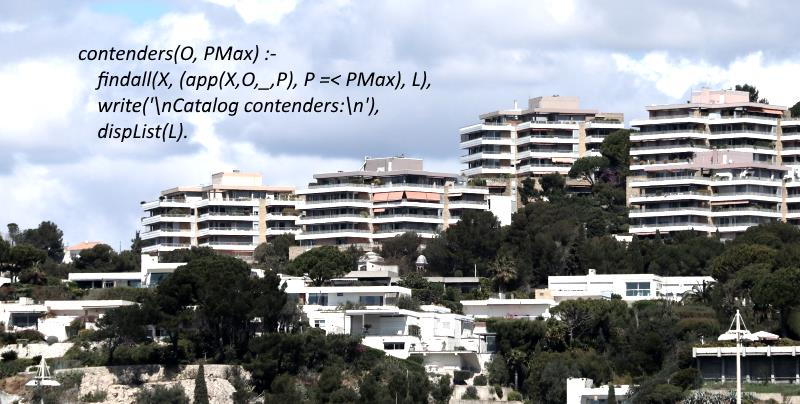# Prolog demo: Looking for an apartment in a catalog

In this demonstration, from an apartment catalog which indicates the price, the number of rooms and the orientation, a search is made to find the optimal product for the given criteria.### Showing the list of apartments

Firstly we enter the catalog in memory, and we add a program that displays the list of products it contains.

``````app(appart1, south, 3, 60).
app(appart2, north, 5, 80).
app(appart3, south, 4, 70).
app(appart4, south, 6, 90).
app(appart5, south, 4, 100).
app(appart6, west, 8, 50).

catalog :-
write('Catalog...\n'),
forall(app(X,O,T,P), format("Code ~a orientation ~a T~D price ~D.000 ~n", [X, O, T, P])).``````

The forall function of prolog is a unification function. The first term is the condition, this condition is confronted with each predicate of type "app" in memory. The second term is the action, it is executed for each occurrence that fulfills the condition.
Since the predicate values are not assigned, the condition is true for all predicates in the database. If we had given "south" as a second value, the condition would have been verified only for apartments with "south" orientation.

So we use this condition without predefined value to display the list of all apartments, regardless of their orientation.

### Knowing apartments that meet criteria

We want to select apartments with a given orientation and a given maximum price.
If it wanted only the apartments with a given orientation and price, it would be simple, we would use the previous catalog rule with two parameters:

``catalog(O,P) :-    write('Catalog...\n'),    forall(app(X,O,T,P), format("Code ~a  T~D  ~n", [X, T])).``

And we would make a request such as this for example:

``catalog(south, 70).``

But we must compare prices with a ceiling value, and the code will be a little more complicated.

The findall function of prolog creates a list of elements that satisfy a condition.

``findall(element, condition involving the element, list) ``

The condition in our case is to have an orientation equal to the given value O, and a price P lower than the price PMax given.

``````contenders(O, PMax) :-
findall(X, (app(X,O,_,P), P =< PMax), L),
write('\nContenders in catalog:\n'),
dispList(L).``````

We group between parenthesis app(X, O, _, P) and the price comparison P =< PMax, to form a condition in a single parameter.

This code returns the L list of all X elements that satisfy the condition. We will see now how to display this list.

### Displaying a list in Prolog

An argument of form [A | B] designates the first element of a list followed by all the other elements. A rule is called with a list L in parameter and prolog dissociates this list into two parts.

It is convenient to list the contents of a list by a recursion. Just replace the [A | B] parameter with B when the rule invokes itself.

``dispList([A|B]) :-  (app(X,_,T,P), X = A),format('~w T~D ~D.000 dollars ~n', [X, T, P]),  dispList(B).``

The dispList rule recursively invokes itself, each time replacing the list with the second part B which does not include the first element. The first element A is treated in the rule.

Our rule unifies element A with the predicate app whose element A is one of the values. The format function displays the values of the predicate that is found.

### Finding the largest apartment that meets the criteria

We are looking for apartment X with a number of rooms. We want the number of rooms to be as large as possible. Other conditions are added.

The not provable operator \+ returns true if its argument is not provable, and false if it is proved.

``\+ (term)``

The interest here is to obtain the unification of successive values by assigning the maximum value to T.

X and T variables are not assigned when invoking the rule, unlike ORT and PMax. They are assigned each time that in a predicate app a value T2 is greater than T and that the other two criteria are respected.

``````largest(X, ORT, PMax) :-
app(X, _, T, _),
\+ (app(_, O, T2, P), (T2 > T, O = ORT, P =< PMax)).``````

The not provable formula looks for an app predicate where the value O is equal to a given ORT value, the value P is less than or equal to a given value PMax, and the value T2 is greater than the value T of the apartment already found.
As long as there is a T2 value greater than T, the formula is proven, there is failure and the unification continues in search of resolution.
As soon as T2 reaches the maximum value, there is success, the resolution is obtained and we return to the point of invocation of the rule with the variables X and T assigned. The apartment matching the criteria is found.

### Looking for an apartment, the complete demo

``````app(appart1, south, 3, 60).
app(appart2, north, 5, 80).
app(appart3, south, 4, 70).
app(appart4, south, 6, 90).
app(appart5, south, 4, 100).
app(appart6, west, 8, 50).

catalog :-
write('Catalog...\n'),
forall(app(X,O,T,P), format("Code ~a orientation ~a T~D price ~D.000 ~n", [X, O, T, P])).

dispList([]).
dispList([A|B]) :-
(app(X,_,T,P), X = A),format('~w T~D ~D.000 dollars ~n', [X, T, P]),
dispList(B).

largest(X, ORT, PMax) :-
app(X, _, T, _),
\+ (app(_, O, T2, P), (T2 > T, O = ORT, P =< PMax)).

best(O,P) :-
largest(X, O, P),
write('\nLargest: '),
write(X).

contenders(O, PMax) :-
findall(X, (app(X,O,_,P), P =< PMax), L),
write('\nContenders in catalog:\n'),
dispList(L).

search(O, P):-
best(O, P);
write('Nothing in catalog.').

test1 :- contenders(south, 80).

test2 :- search(south, 80).``````

To use this program, use the consult command from the prolog console. Then type:

``````catalog.
test1.
test2.``````

For respectively, view the list of all apartments, the list of apartments facing south and price less than 80, finally the largest among those who meet these criteria.

You can also give your own criteria, as in this example:

``search(north, 100).``